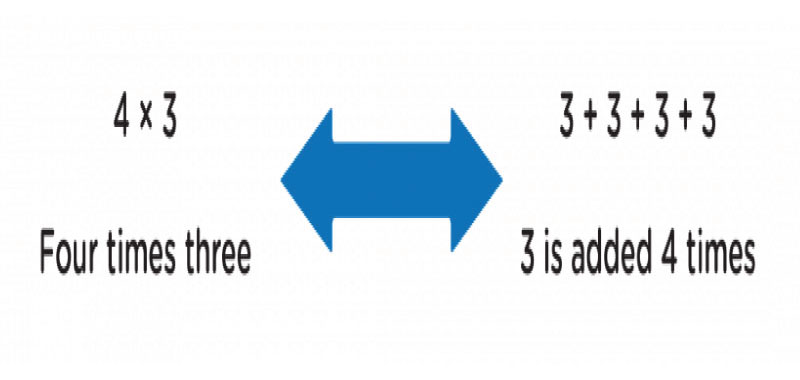# Mastering Multiplication: Tips and Tricks for Speed and Accuracy

Author: Nidhita

## Multiplication: Tips and Tricks for Speed and Accuracy

Multiplication is a fundamental skill that forms the foundation for many mathematical concepts. Mastering multiplication is crucial, whether you're a student learning basic arithmetic or an adult looking to enhance your mental math abilities. This article will explore tips to help you improve your speed and accuracy when dealing with multiplication tables. By incorporating these strategies into your practice routine, you'll soon multiply with ease and confidence.### Understand the Multiplication Tables:

The multiplication tables, also known as times tables, are the building blocks of multiplication. These tables provide a quick reference for the products of numbers from 1 to 10. To master it, it's essential to memorize these tables. Start by focusing on one table at a time and practicing reciting the products aloud or writing them down. For example, for the seven times table, you should memorize the sequence: 7, 14, 21, 28, 35, 42, 49, 56, 63, and 70.

### Visualize Multiplication:

Visualization can be an assertive tool for understanding and recalling multiplication facts —picture multiplication as groups of objects or arrays. For instance, when multiplying four by 3, visualize four groups, each with three objects. This mental image will help you grasp the concept and make it easier to recall the product. Additionally, visualizing can aid in solving more complex problems by breaking them down into simpler, manageable parts.

### Break Down Larger Numbers:

Multiplying large numbers can be daunting, but breaking them down into smaller, more manageable parts can simplify the process. For example, if you need to multiply 47 by 6, you can break it down into (40 × 6) + (7 × 6). Multiply each part separately and add the results together: 240 + 42 = 282. Breaking down more significant numbers not only makes the calculation more manageable but also reduces the chance of errors.

### Take Advantage of Known Facts:

Leveraging a known multiplication chartand facts can significantly speed up your calculations. For instance, if you know that seven times 8 equals 56, you can quickly determine that 70 times 8 equals 560. By recognizing patterns and relationships between numbers, you can efficiently apply your knowledge to solve more complex problems. Practice identifying these connections between facts, and you'll multiply numbers faster than ever.

### Apply Mental Math Techniques:

Mental math techniques can be beneficial when multiplying numbers. Here are a few techniques to consider: Multiplying by powers of 10: When multiplying a number by a power of 10, shift the digits of the number to the left by the number of zeros in the power of 10. For example, 25 × 1,000 = 25,000 (shift three places to the left).

Multiplying by 9: To multiply a number by 9, multiply it by ten and subtract the original number. For example, 9 × 7 = (10 × 7) - 7 = 70 - 7 = 63.

Distributive Property: The distributive property states that multiplying a number by a sum is the same as multiplying the number by each addend and then adding the results. For example, multiplying 17 by five can break it down as (10 × 5) + (7 × 5) = 50 + 35 = 85.

### Conclusion

Mastering multiplication is a valuable skill that can benefit individuals of all ages. Implementing the tips and tricks outlined above can enhance your abilities and help you achieve incredible speed and accuracy. Remember, the key to mastering anything is practice. Dedicate regular time to reviewing the multiplication tables and incorporating visualization techniques into your learning process. Break down more significant numbers into manageable parts and leverage your knowledge of known facts to simplify calculations.